# Voltage/Current Converter OpAmp Circuits

• #### Question 1

 Don’t just sit there! Build something!!

Learning to mathematically analyze circuits requires much study and practice. Typically, students practice by working through lots of sample problems and checking their answers against those provided by the textbook or the instructor. While this is good, there is a much better way.

You will learn much more by actually building and analyzing real circuits, letting your test equipment provide the “answers” instead of a book or another person. For successful circuit-building exercises, follow these steps:

1. Carefully measure and record all component values prior to circuit construction.
2. Draw the schematic diagram for the circuit to be analyzed.
3. Carefully build this circuit on a breadboard or other convenient medium.
4. Check the accuracy of the circuit’s construction, following each wire to each connection point, and verifying these elements one-by-one on the diagram.
5. Mathematically analyze the circuit, solving for all voltage and current values.
6. Carefully measure all voltages and currents, to verify the accuracy of your analysis.
7. If there are any substantial errors (greater than a few percent), carefully check your circuit’s construction against the diagram, then carefully re-calculate the values and re-measure.

Avoid using the model 741 op-amp, unless you want to challenge your circuit design skills. There are more versatile op-amp models commonly available for the beginner. I recommend the LM324 for DC and low-frequency AC circuits, and the TL082 for AC projects involving audio or higher frequencies.

As usual, avoid very high and very low resistor values, to avoid measurement errors caused by meter “loading”. I recommend resistor values between 1 kΩ and 100 kΩ.

One way you can save time and reduce the possibility of error is to begin with a very simple circuit and incrementally add components to increase its complexity after each analysis, rather than building a whole new circuit for each practice problem. Another time-saving technique is to re-use the same components in a variety of different circuit configurations. This way, you won’t have to measure any component’s value more than once.

• #### Question 2

Calculate the current through resistor R2 in this opamp circuit for several different values of R2:R2 IR2 1 kΩ 2 kΩ 3 kΩ 4 kΩ 5 kΩ 6 kΩ

For each value of R2, what is it that establishes the amount of current through it? Do you see any practical value for a circuit such as this?

• #### Question 3

Explain how the operational amplifier maintains a constant current through the load:Write an equation solving for the regulated load current, given any relevant variables shown in the schematic diagram (R1, VZ, Vsupply, AV(OL), etc.).

• #### Question 4

Explain how the operational amplifier maintains a constant current through the load:Write an equation solving for the regulated load current, given any relevant variables shown in the schematic diagram (R1, VZ, Vsupply, AV(OL), etc.). Also, describe what would have to be changed in this circuit in order to set the regulated current at a different value.

• #### Question 5

At first glance, the feedback appears to be wrong in this current-regulating circuit. Note how the feedback signal goes to the operational amplifier’s non-inverting ( ) input, rather than the inverting input as one would normally expect for negative feedback:Explain how this op-amp really does provide negative feedback, which of course is necessary for stable current regulation, as positive feedback would be completely unstable.

• #### Question 6

Predict how the operation of this current regulator circuit will be affected as a result of the following faults. Consider each fault independently (i.e. one at a time, no multiple faults):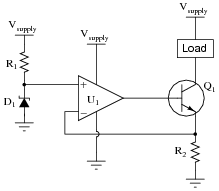Resistor R1 fails open:
Zener diode D1 fails shorted:
Resistor R2 fails open:
Zener diode D1 fails open:
Wire between opamp output and transistor base breaks open:

For each of these conditions, explain why the resulting effects will occur.

• #### Question 7

Predict how the operation of this current regulator circuit will be affected as a result of the following faults. Consider each fault independently (i.e. one at a time, no multiple faults):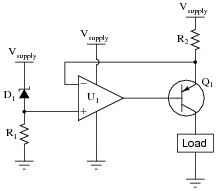Resistor R1 fails open:
Resistor R2 fails open:
Solder bridge (short) across resistor R2:
Zener diode D1 fails shorted:
Zener diode D1 fails open:
Wire between opamp output and transistor base breaks open:

For each of these conditions, explain why the resulting effects will occur.

• #### Question 8

The simplest electronic device capable of converting a current signal into a voltage signal is a resistor: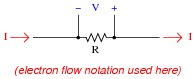Precision resistors typically work very well for this purpose, especially when the amount of voltage dropped across it is of little consequence. This is why shunt resistors are frequently used in power circuitry to measure current, a low-resistance “shunt” resistance element dropping voltage in precise proportion to the current going through it.

However, if we cannot afford to drop any voltage across a resistance in the circuit, this technique of current-to-voltage conversion will not be very practical. Consider the following scientific apparatus, used to measure the photoelectric effect (electrons emitted from a solid surface due to light striking it):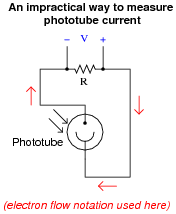The current output by such a phototube is very small, and the voltage output by it is smaller yet. If we are to measure current through this device, we will have to find some way other than a shunt resistor to do it.

Enter the operational amplifier, to the rescue! Explain how the following opamp circuit is able to convert the phototube’s weak current signal into a strong voltage signal, without imposing any significant resistance into the phototube circuit: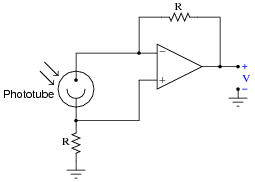• #### Question 9

Shown here is a simple circuit for constructing an extremely high input impedance voltmeter on a wireless breadboard, using one half of a TL082 dual op-amp: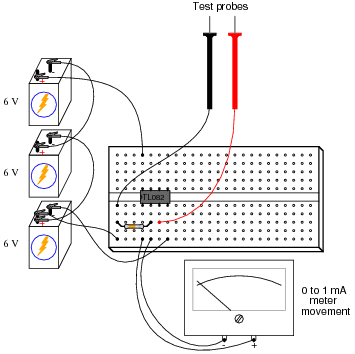Draw a schematic diagram of this circuit, a calculate the resistor value necessary to give the meter a voltage measurement range of 0 to 5 volts.